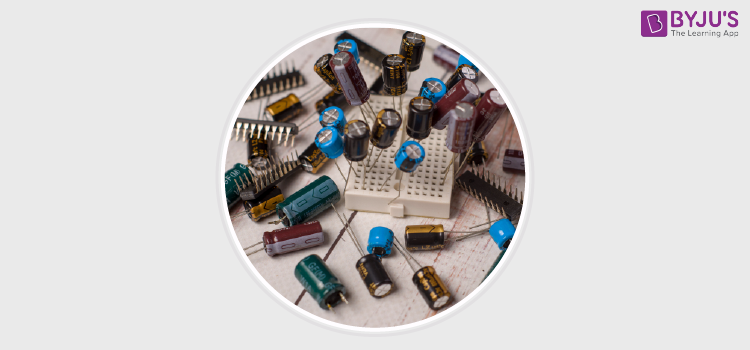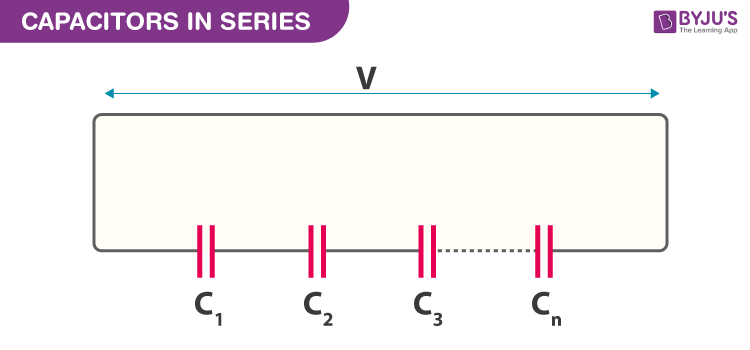Win up to 100% scholarship on Aakash BYJU'S JEE/NEET courses with ABNAT Win up to 100% scholarship on Aakash BYJU'S JEE/NEET courses with ABNAT

# Capacitors in Series

A Capacitor is a two terminal passive electrical component whose function is to store energy electrostatically in an electric field. The different forms of capacitor vary widely but all contain two electrical conductors separated by a dielectric.The conductors can either be an aluminium foil or disks, thin films of metal, etc. The dielectric acts to increase the capacitor’s charge capacity. A dielectric can be ceramic, glass, plastic film, air, paper, mica, etc. The basic function of a capacitor is to hold a group of electrons. Unlike a resistor, a capacitor does not dissipate energy. Instead, a capacitor stores energy in the form of an electrostatic field between its plates. The increase in the size of plates increases the capacitance as there is more space for the electrons to come out.

## Series Combination of Capacitors

Several capacitors may be connected together in a variety of applications. Multiple connections of capacitors act as a single equivalent capacitor. The capacitance of the resultant capacitor depends on both individual capacitors and the way they are connected. The two common types of connections are called series and parallel. In this section, we will be discussing the series combination of capacitors.

When the capacitors are connected in the form of a series combination, the capacitance in total is less than the individual capacitances of the series capacitors. If one, two or a number of capacitors are connected in the series form, the overall effect is the single or equivalent capacitor, which has the total sum of the spacings between the plates of the individual capacitors. The increase in the plate spacing results in the decreased capacitance, with all the other factors remaining unchanged.The series combination of capacitors is as shown in the above figure. Thus, the total capacitance of the series combination of capacitors is less than the individual capacitances of the capacitors. The formula to calculate the total capacitance of the series combination capacitors is in the same form as that for calculating the parallel combination of resistances. The figure below shows the formula to calculate the total capacitance of capacitors connected in series.

### Capacitors in Series Equation

When adding the series capacitors, the reciprocal ( 1/C ) of all the individual capacitors are added together (just like the resistors in the parallel combination), instead of the capacitances themselves. The total value of the capacitors in the form of series combination equals the reciprocal of the sum of the reciprocal of their individual capacitances.

Remember in the series combination circuit of the capacitor, there is only one path for the flow of electric current. The number of electrons discharging from the bottom will have the same number of electrons from the top, thus increasing the capacitance.

Thus, in the series circuit, the space between the capacitors are farther apart as the space between the plates of two capacitors adds together.

### Capacitors in Series Formula

 Series capacitance $$\begin{array}{l}C_{total}=\frac{1}{\frac{1}{C_{1}}+\frac{1}{C_{2}}+…..\frac{1}{C_{n}}}\end{array}$$ Capacitors in Series Equation $$\begin{array}{l}\frac{1}{C_{T}}=\frac{1}{C_{1}}+\frac{1}{C_{2}}+\frac{1}{C_{3}}+…..etc\end{array}$$

#### Capacitors in Series Video Lesson## Frequently Asked Questions on Capacitors in Series

Q1

### Give any two uses of capacitors.

To store electric charge.
In AC circuit to control current.

Q2

### What is the formula for the effective capacitance of a number of capacitors in series?

If a number of capacitors of capacitance C1, C2, C3 ……..Cn are connected in series and if Cs is the effective capacitance then
(1/C1) + (1/C2) + (1/C3) ——–(1/Cn)

Q3

### What form of energy is stored in a charged capacitor?

Energy is stored as electric potential energy in a charged capacitor.

Q4

### What is the SI unit of capacitance of a capacitor?

Farad is the SI unit for capacitance.